This document contains R versions of the boxed examples from Chapter 4 of the Analysis and Interpretation of Freshwater Fisheries Data book. Some sections build on descriptions from previous sections, so each section may not stand completely on its own. More thorough discussions of linear models; type-I, II, and III sums-of-squares, and least-squares means are found in the preliminaries vignette.

The following additional packages are required to complete all of the examples (with the required functions noted as a comment and also noted in the specific examples below).

> library(FSA)          # fitPlot, Subset
> library(car)          # Anova, durbinWatsonTest, vif
> library(Hmisc)        # rcorr
> library(Kendall)      # kendall
> library(lsmeans)      # lsmeans
> library(multcomp)     # glht, mcp, cld
> library(nlstools)     # overview, nlsBoot
> library(plotrix)      # thigmophobe.labels, rescale

In addition, external tab-delimited text files are used to hold the data required for each example. These data are loaded into R in each example with read.table(). Before using read.table() the working directory of R must be set to where these files are located on your computer. The working directory for all data files on my computer is set below.

> setwd("c:/aaaWork/web/fishR/BookVignettes/AIFFD/")

I also prefer to not show significance stars for hypothesis test output and set contrasts in such a manner as to force R output to match SAS output for linear model summaries. These options are set below.

> options(show.signif.stars=FALSE,contrasts=c("contr.sum","contr.poly"))

Finally, I set the random number seed so that the boostrapping results can be repeated.

> set.seed(983452)

## 4.1 Log-Linear Model to Test for Year-Class Abundance Differences

Below we conduct a test for year-class abundance differences among the 1990 to 1997 year-classes (yearcl) based on catch rates of age-0, age-1, and age-2 crappies (Pomoxis spp.) (age in years) from Weiss Lake (Table 4.1 in text). Trap-net catch rates (catch) were transformed to natural log values to homogenize variances as recommended by Kimura (1988) for log-linear analysis. The data in Table 4.1 were rearranged to conduct the analysis. Year of collection (yearcol) was included in the data file, and the following R code was used to conduct the analysis.

### 4.1.1 Preparing Data

The box4_1.txt is read, the structure of the data frame is observed, and a new variable, lcatch, that is the natural log of the catch variable is created.

> d1 <- read.table("data/box4_1.txt",header=TRUE)
> str(d1)
'data.frame':   24 obs. of  4 variables:
$yearcol: int 1990 1991 1992 1991 1992 1993 1992 1993 1994 1993 ...$ yearcl : int  1990 1990 1990 1991 1991 1991 1992 1992 1992 1993 ...
$age : int 0 1 2 0 1 2 0 1 2 0 ...$ catch  : num  8.03 5.32 2.43 0.47 0.39 0.39 0.61 0.97 0.61 1.38 ...
> d1$lcatch <- log(d1$catch)

In addition, R must be told explicitly that age and yearcl are group factor rather than numeric variables.

> d1$age <- factor(d1$age)
> d1$yearcl <- factor(d1$yearcl)

### 4.1.2 Two-Way ANOVA Model

The authors of the box fit a two-way ANOVA withOUT an interaction term. While a two-way ANOVA is generally fit with an interaction term, not using an interaction term is appropriate here because an interaction cannot be estimated as there are not multiple observations for each combination of the two factors. This fact is best illustrated with a two-way frequency table constructed from the two group factor variables with xtabs().

> xtabs(~age+yearcl,data=d1)
   yearcl
age 1990 1991 1992 1993 1994 1995 1996 1997
0    1    1    1    1    1    1    1    1
1    1    1    1    1    1    1    1    1
2    1    1    1    1    1    1    1    1

The two-way ANOVA model without the interaction term is fit with lm(). The ANOVA table using type-III SS is then extracted from the lm() object with Anova() from the car package. From this, there is evidence for a significant difference in means among ages and among year-classes.

> lm1 <- lm(lcatch~age+yearcl,data=d1)
> Anova(lm1,type="III")
             Sum Sq     Df F value    Pr(>F)
(Intercept)  2.7457  1.000 12.4839  0.003309
age          4.0952  2.000  9.3097  0.002683
yearcl      19.0150  7.000 12.3508 4.994e-05
Residuals    3.0792 14.000
Total       24.0000 28.935                  

### 4.1.3 Least-Squares Means for Year-Class

Least-squares means are computed with lsmeans() from the lsmeans package using a right-hand-sided formula as the second argument to isolate the least-square means for each factor variable. From this, it appears that CPE generally decreases (not surprisingly) with age and that 1990 and 1996 are relatively strong year-classes and 1991 is a relatively poor year-class.

> lsmeans(lm1,~age)
 age     lsmean        SE df   lower.CL  upper.CL
0    0.6973961 0.1658088 14  0.3417717 1.0530205
1    0.5576609 0.1658088 14  0.2020365 0.9132853
2   -0.2403432 0.1658088 14 -0.5959676 0.1152812

Results are averaged over the levels of: yearcl
Confidence level used: 0.95 
> lsmeans(lm1,~yearcl)
 yearcl     lsmean        SE df    lower.CL   upper.CL
1990    1.5475164 0.2707646 14  0.96678413  2.1282486
1991   -0.8794132 0.2707646 14 -1.46014545 -0.2986810
1992   -0.3396840 0.2707646 14 -0.92041618  0.2410483
1993    0.6259558 0.2707646 14  0.04522358  1.2066880
1994    0.6280314 0.2707646 14  0.04729921  1.2087637
1995   -0.2701080 0.2707646 14 -0.85084020  0.3106243
1996    1.7311522 0.2707646 14  1.15041997  2.3118844
1997   -0.3375473 0.2707646 14 -0.91827955  0.2431849

Results are averaged over the levels of: age
Confidence level used: 0.95 

### 4.1.4 Multiple Comparisons

The authors of the box use Fisher’s LSD multiple comparison procedure. In general, this procedure does not guard well against an inflated experimentwise error rate. In general, Tukey’s HSD procedure performs better in this regard and will be illustrated below.

Tukey’s multiple comparison procedure, implemented through glht() from the multcomp package, can be used to identify where the differences in means occur. The glht() function requires the lm() object as the first argument. The second argument is also required and uses mcp() to declare a “multiple comparison procedure.” In this instance the argument to mcp() is the factor variable in the lm() object for which you are testing for differences set equal to the "Tukey" string. The result from glht() is saved to an object that can be submitted to summary() to extract p-values for each difference in pairs of means, to confint() to extract confidence intervals for each difference in pairs of means, and to cld() to identify significance letters that depict significant differences among means.

> mc1a <- glht(lm1,mcp(age="Tukey"))
> summary(mc1a)
           Estimate Std. Error t value Pr(>|t|)
1 - 0 == 0  -0.1397     0.2345  -0.596  0.82457
2 - 0 == 0  -0.9377     0.2345  -3.999  0.00348
2 - 1 == 0  -0.7980     0.2345  -3.403  0.01113
> mc1yc <- glht(lm1,mcp(yearcl="Tukey"))
> summary(mc1yc)
Warning in RET$pfunction("adjusted", ...): Completion with error > abseps Warning in RET$pfunction("adjusted", ...): Completion with error > abseps
                  Estimate Std. Error t value Pr(>|t|)
1991 - 1990 == 0 -2.426930   0.382919  -6.338  < 0.001
1992 - 1990 == 0 -1.887200   0.382919  -4.928  0.00417
1993 - 1990 == 0 -0.921561   0.382919  -2.407  0.30867
1994 - 1990 == 0 -0.919485   0.382919  -2.401  0.31101
1995 - 1990 == 0 -1.817624   0.382919  -4.747  0.00563
1996 - 1990 == 0  0.183636   0.382919   0.480  0.99959
1997 - 1990 == 0 -1.885064   0.382919  -4.923  0.00408
1992 - 1991 == 0  0.539729   0.382919   1.410  0.83898
1993 - 1991 == 0  1.505369   0.382919   3.931  0.02440
1994 - 1991 == 0  1.507445   0.382919   3.937  0.02412
1995 - 1991 == 0  0.609305   0.382919   1.591  0.74834
1996 - 1991 == 0  2.610565   0.382919   6.818  < 0.001
1997 - 1991 == 0  0.541866   0.382919   1.415  0.83650
1993 - 1992 == 0  0.965640   0.382919   2.522  0.26162
1994 - 1992 == 0  0.967715   0.382919   2.527  0.25991
1995 - 1992 == 0  0.069576   0.382919   0.182  1.00000
1996 - 1992 == 0  2.070836   0.382919   5.408  0.00171
1997 - 1992 == 0  0.002137   0.382919   0.006  1.00000
1994 - 1993 == 0  0.002076   0.382919   0.005  1.00000
1995 - 1993 == 0 -0.896064   0.382919  -2.340  0.33844
1996 - 1993 == 0  1.105196   0.382919   2.886  0.14944
1997 - 1993 == 0 -0.963503   0.382919  -2.516  0.26428
1995 - 1994 == 0 -0.898139   0.382919  -2.346  0.33553
1996 - 1994 == 0  1.103121   0.382919   2.881  0.15063
1997 - 1994 == 0 -0.965579   0.382919  -2.522  0.26189
1996 - 1995 == 0  2.001260   0.382919   5.226  0.00229
1997 - 1995 == 0 -0.067439   0.382919  -0.176  1.00000
1997 - 1996 == 0 -2.068700   0.382919  -5.402  0.00180
> cld(mc1yc)
Warning in RET$pfunction("adjusted", ...): Completion with error > abseps 1990 1991 1992 1993 1994 1995 1996 1997 "c" "a" "ab" "bc" "bc" "ab" "c" "ab"  An plot of the group means, with appropriate confidence intervals is constructed with fitPlot() from the FSA package. The significance letters can be added to the means plot with addSigLetters() from the NCStats package. See ?addSigLetters for a description of the arguments. > fitPlot(lm1,which="yearcl",xlab="Year Class",ylab="Loge(Catch)",main="") > addSigLetters(lm1,which="yearcl",lets=c("c","a","ab","bc","bc","ab","c","ab"), pos=c(4,2,2,2,4,2,2,2),cex=0.75)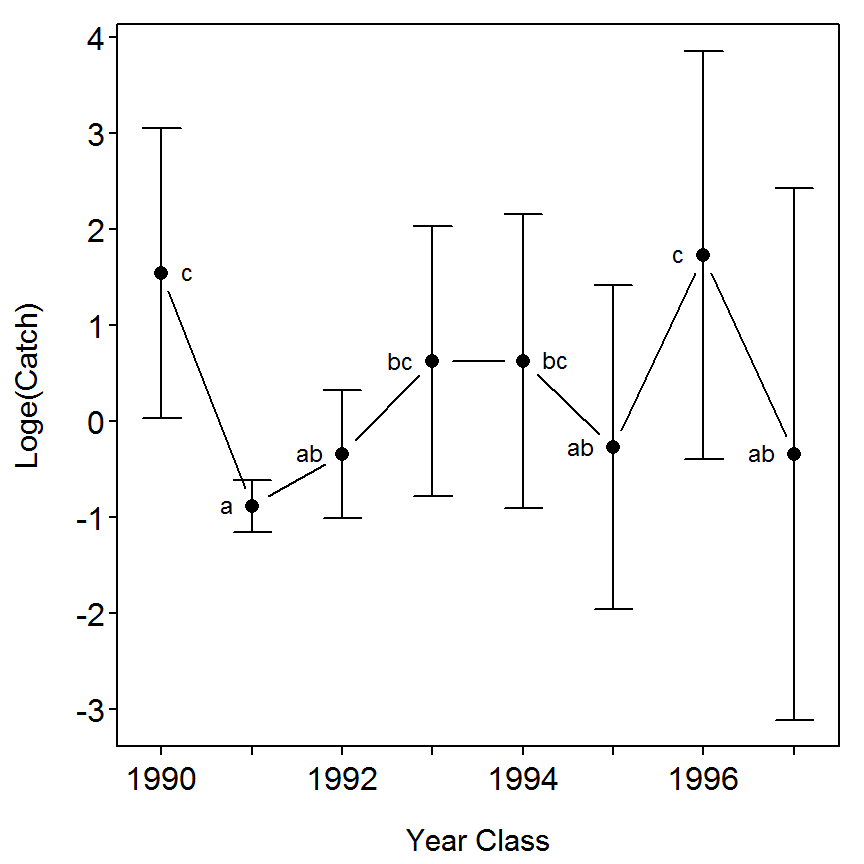## 4.3 Evaluation of Spatial Differences in Recruit Abundance Table 4.2 (in text) contains a data set to test for spatial differences in age-0 largemouth bass (Micropterus salmoides) catch in Lake Normandy, Tennessee (data from Sammons and Bettoli (2000)). In this example, four distinct areas of the reservoir (Lower Basin [LB], Riley Creek [RC], Upper Basin [UB], and Carroll Creek [CC]) were chosen to examine spatial variation in abundance of fish along 100-m shoreline electrofishing transects. A handheld DC electrofishing unit was used at night. Six fixed sites, or replicate transects, were chosen within each area and sampled three times at 2-week intervals starting the second week of August 1992 and ending the second week of September 1992. Thus, 24 transects were conducted over three time intervals for a total of 72 transects, or observations. Because replicate samples were collected at fixed locations over the three time periods within each of the same areas, a split-plot repeated-measured ANOVA was used to test for differences in number of fish among areas (Maceina et al. 1994). In addition, this analysis also tested for differences in catch over time and examined the time x area interaction. The code and analysis were divided into main-plot A, which included the class variables area, replicates (rep), and the area x rep interaction, and subplot B, which contained the time and the time x area interaction effects. The mean square error (MS, or type III sums of squares) of the area x rep term was used as the error term in the denominator and the MS error for area as the numerator of an F-test to determine if statistical differences in the number caught among the four areas in main-plot A. The MS error generated from the entire ANOVA was used in the denominator of the F-test to determine if statistical differences in catch occurred over the three time periods (subplot B), as well as for testing for any interaction between time periods and areas (subplot B). The following R code provides output to test for differences in catch among areas. ### 4.3.1 Preparing Data The box4_3.txt data file is read and the structure of the data frame is observed below. The rep and time variables are converted to group factor variables for the analysis. > d3 <- read.table("data/box4_3.txt",header=TRUE) > str(d3) 'data.frame': 72 obs. of 6 variables:$ year : int  92 92 92 92 92 92 92 92 92 92 ...
$month: int 8 8 8 8 8 8 8 8 8 8 ...$ area : Factor w/ 4 levels "CC","LB","RC",..: 1 1 1 1 1 1 2 2 2 2 ...
$rep : int 1 2 3 4 5 6 1 2 3 4 ...$ time : int  1 1 1 1 1 1 1 1 1 1 ...
$count: int 0 3 0 0 2 1 4 2 11 3 ... > d3$rep <- factor(d3$rep) > d3$time <- factor(d3$time) ### 4.3.2 Helper Function As is demonstrated in the box, the error term for the “plot” term uses the error term associated with the “plot” and “treatment” interaction term. As far as I know R does not have a built-in function for computing F-tests with other than the residual or error MS from the full model fit. Thus, the ANOVA table for these terms must be built by hand by extracting the appropriate MS and df from the Type-III ANOVA table. This hand calculation is simply finding the appropriate values in the ANOVA table using numerical and named subscripts. The following function is a helper function that does the “hand” calculations to create the appropriate F-tests. It should be noted that this function only works if the “plot” and “treatment” are the first two terms in the model and their interactions is the third term. Fitting models in that order is demonstrated below. > rmsp2 <- function(object,type=c("III","II","I")) { type <- match.arg(type) # extract df and SS of appropriate rows of ANOVA table if (type=="I") { res <- anova(object)[1:2,1:3] } else if (type=="III") { res <- Anova(object,type=type)[2:4,2:1] } else { res <- Anova(object,type=type)[1:3,2:1] } # compute MSs res[,"Mean Sq"] <- res[,2]/res[,1] # MS in third position is the error MS errorMS <- res[3,"Mean Sq"] # compute F for first two positions (put NA in last position) res[,"F"] <- c(res[1:2,"Mean Sq"]/errorMS,NA) # convert Fs to p-values res[,"PR(>F)"] <- c(pf(res[1:2,"F"],res[1:2,"Df"],res[3,"Df"],lower.tail=FALSE),NA) res } ### 4.3.3 Fitting The Model The repeated-measures split-plot ANOVA can be fit using lm() with a twist. The twist is that terms() must be used to control the order that the model terms will be fit. This is important because the “plot” and “treatment” terms must be fit first followed by their interaction and then followed by the subplot terms. This function basically has the explicit model formula as the first argument and then the keep.order=TRUE argument so that R does not put all of the interactions terms at the end of the model formula. The ANOVA table to match the output in the box uses Anova() and type="II" (despite the fact that the table in the box is listed as having used type-III SS). > lm1 <- lm(terms(count~area+rep+area*rep+time+time*area,keep.order=TRUE),data=d3) > Anova(lm1,type="II")  Sum Sq Df F value Pr(>F) area 65.264 3.00 8.4667 0.0001781 rep 21.403 5.00 1.6659 0.1651616 area:rep 43.319 15.00 1.1240 0.3677616 time 18.361 2.00 3.5730 0.0373519 area:time 14.861 6.00 0.9640 0.4617672 Residuals 102.778 40.00 Total 71.000 265.99  The hypothesis tests that use the area:rep mean square as the error term are then computed with the rsmp2() helper function defined above. > rmsp2(lm1)  Df Sum Sq Mean Sq F PR(>F) area 3 65.264 21.7546 7.5329 0.00265 rep 5 21.403 4.2806 1.4822 0.25351 area:rep 15 43.319 2.8880 Total 23 129.986  ### 4.3.4 Multiple Comparisons The authors of the box use the Student-Newman-Keuls’ (SNK) multiple comparison procedure. The SNK method can provide more power than Tukey’s HSD method but it tends not to control the experimentwise error rate at the desired level and, because it works in a sequential fashion, it does not produce appropriate confidence intervals (see this and Hsu (1996)). Thus, in general, Tukey’s HSD procedure performs better than SNK and will be illustrated below. Tukey’s multiple comparison procedure is implemented with glht() as described in Box 4.1. In this example, the model was refit without the time:area interaction because this term was insignificant as shown in the analysis above and its inclusion in the model causes problems when examining the multiple comparison procedures for just time. Following the model refit below, the remaining commands below tell R to perform a Tukey multiple comparison procedure test on the time variable in the lm1 model. > lm1a <- lm(terms(count~area+rep+area*rep+time,keep.order=TRUE),data=d3) > mc1 <- glht(lm1a,mcp(time="Tukey")) > summary(mc1)  Estimate Std. Error t value Pr(>|t|) 2 - 1 == 0 -0.8333 0.4616 -1.805 0.1792 3 - 1 == 0 -1.2083 0.4616 -2.617 0.0315 3 - 2 == 0 -0.3750 0.4616 -0.812 0.6974 > cld(mc1)  1 2 3 "b" "ab" "a"  I have not yet figured out how to perform the multiple comparisons using an error term other than the residual or error MS. ## 4.4 The Use of Catch-Curve Regression to Identify Weak and Strong Year-Class Formation This example contains a data set (data published in Maceina and Bettoli (1998)) that uses catch-curve regression to detect strong and weak year-class formation in a largemouth bass (Micropterus salmoides) population. In addition, a reservoir hydrologic variable is included that will be used later (see section 4.3.4 in the text) to examine the association between year-class strength and an environmental variable. In spring 1993, 653 age-2 to age-11 largemouth bass were collected using DC electrofishing. Age-length keys (Bettoli and Miranda 2001) were used to estimate the age structure for the entire sample from examination of 190 otoliths. The R code below first computes the regression between the natural log of number at age (lnum) against age and uses the predicted values for the natural log of number at age (plnum) as weighting factors when the catch-curve analysis was recomputed. Thus, the second catch-curve regression computes the least-squares fit using the predicted values from the first fit as weights. From this regression, the residuals were computed and printed with the year-class (yearcl) and age identified. For this analysis, it was assumed that all fish age-2 and older were fully recruited to the electrofishing gear and the fishery. This analysis is extended in Box 4.6. ### 4.4.1 Preparing Data The box4_4.txt data file is read and the structure of the data frame is observed below. The authors of the box computed the natural log number of fish caught (they also added 1 before taking the logarithm to adjust for catches with zero fish) and the common logarithm [I am not sure why they switched to common logarithms here] of the mean retention time between April and July. > d4 <- read.table("data/box4_4.txt",header=TRUE) > str(d4) 'data.frame': 10 obs. of 4 variables:$ yearcl : int  91 90 89 88 87 86 85 84 83 82
$age : int 2 3 4 5 6 7 8 9 10 11$ num    : int  175 273 28 79 18 49 21 8 0 2

### 4.4.3 Catch Curve Analysis II (Using the Weights)

The linear regression between lnum and age using plnum as weights (a weighted catch-curve analysis) is again fit with lm(), but including the weights= argument. The ANOVA table is extracted from the lm() object with anova() and the parameter estimates are extracted with summary() (under the “coefficients” heading), respectively.

> lm2 <- lm(lnum~age,weights=plnum1,data=d4)
> anova(lm2)
          Df Sum Sq Mean Sq F value   Pr(>F)
age        1 47.500  47.500  23.584 0.001262
Residuals  8 16.113   2.014
Total      9 63.613                         
> summary(lm2)
Coefficients:
Estimate Std. Error t value Pr(>|t|)
(Intercept)  6.34469    0.56991  11.133 3.79e-06
age         -0.48052    0.09895  -4.856  0.00126

Residual standard error: 1.419 on 8 degrees of freedom
Multiple R-squared: 0.7467, Adjusted R-squared: 0.715
F-statistic: 23.58 on 1 and 8 DF,  p-value: 0.001262 

### 4.4.4 Identifying Year-Class Strength

Year-class strength is defined in the box as the studentized residuals from the weighted catch-curve analysis. The authors of the box created a table that contained, among other things, the predicted values with corresponding standard errors from the weighted catch curve analysis. These values are computed with predict() when given the lm() object and se.fit=FALSE.

> ( preds <- predict(lm2,se.fit=TRUE) )
$fit 1 2 3 4 5 6 7 8 5.383652 4.903132 4.422612 3.942092 3.461572 2.981052 2.500533 2.020013 9 10 1.539493 1.058973$se.fit
1         2         3         4         5         6         7         8
0.4019946 0.3307134 0.2769905 0.2523032 0.2648977 0.3102667 0.3767503 0.4551888
9        10
0.5404014 0.6296437

$df  8$residual.scale
 1.419179

These values were then combined with the observed ages, year-class designation, and log numbers of fish at each age, and the raw residuals (lm2\$residuals), internally studentized residuals (returned from rstandard()), and Cook’s distance values (returned from cooks.distance()) into a new data.frame using data.frame(). > ycs <- data.frame(age=d4$age, yearcl=d4$yearcl, lnum=d4$lnum, meanret=d4$meanret, lmeanret=d4$lmeanret, plnum=preds$fit, pse=preds$se.fit,
resid=lm2$residuals, sresid=rstandard(lm2), cooksD=cooks.distance(lm2)) > round(ycs,3) # rounded for display purposes only  age yearcl lnum meanret lmeanret plnum pse resid sresid cooksD 1 2 91 5.170 13.7 1.137 5.384 0.402 -0.213 -0.470 0.087 2 3 90 5.613 16.9 1.228 4.903 0.331 0.710 1.306 0.316 3 4 89 3.367 9.6 0.982 4.423 0.277 -1.055 -1.724 0.304 4 5 88 4.382 47.7 1.679 3.942 0.252 0.440 0.658 0.031 5 6 87 2.944 19.5 1.290 3.462 0.265 -0.517 -0.720 0.035 6 7 86 3.912 49.5 1.695 2.981 0.310 0.931 1.209 0.119 7 8 85 3.091 31.0 1.491 2.501 0.377 0.591 0.709 0.051 8 9 84 2.197 9.6 0.982 2.020 0.455 0.177 0.192 0.004 9 10 83 0.000 10.5 1.021 1.539 0.540 -1.539 -1.426 0.254 10 11 82 1.099 23.2 1.365 1.059 0.630 0.040 0.029 0.000 There are two types of “studentized residuals” – internally and externally studentized (or jackknife) residuals. SAS appears to produce internally studentized residuals. The internally studentized residuals are computed in R with rstandard() whereas the externally studentized residuals are computed with rstudent(). The graphic in the box is a bit cumbersome to construct for a couple of reasons. First, the values on the x-axis of the plot are in reverse order. To construct this axis, the observed log numbers of fish are plotted against age, but xaxt="n" will be used to tell R not to construct an x-axis. The x-axis will then be added “manually” by placing year-class labels at the tick-marks where the ages would have been. Second, the vertical lines corresponding to the residuals are constructed manually with a loop. > plot(lnum~age,data=d4,xlab="Year Class (19__)",xaxt="n", ylab="loge Number-at-Age",pch=19) > axis(1,at=ycs$age,labels=ycs$yearcl) # Add 'new' x-axis > abline(lm2,lwd=2) # Add regression line > for (i in 1:nrow(ycs)) { # Add residual lines with(ycs,lines(c(age[i],age[i]),c(lnum[i],plnum[i]),lty=2,lwd=2)) }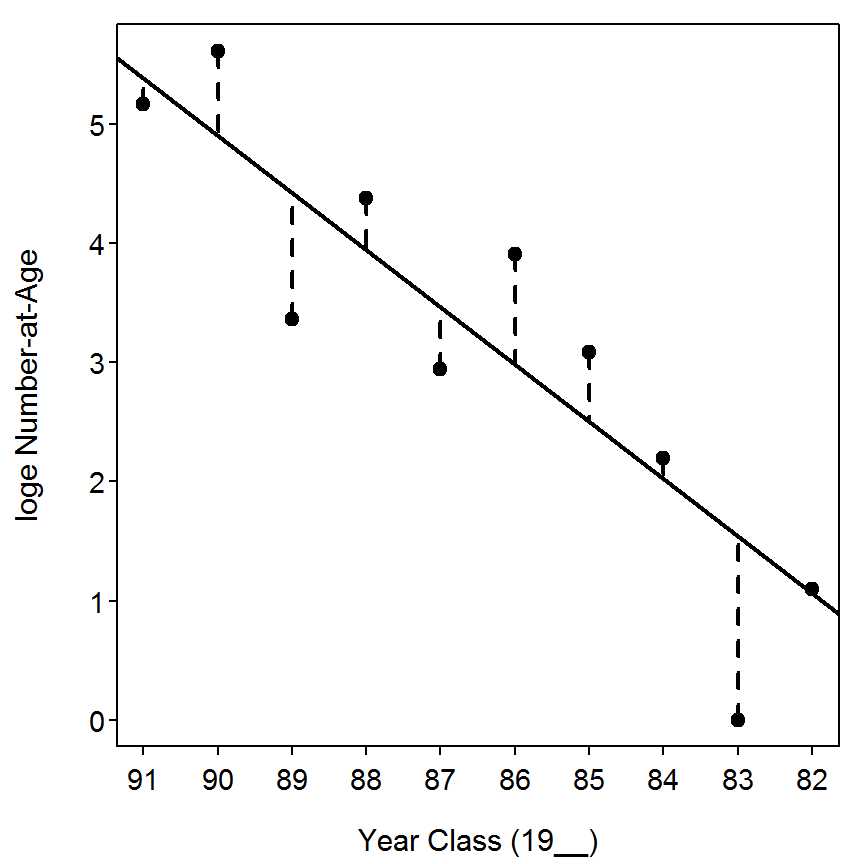There are many other ways to show the year-class strength (i.e., studentized residuals). The two plots below are examples, > plot(sresid~yearcl,data=ycs,type="b",xlab="Year Class (19__)", ylab="Studentized Residual", pch=19,ylim=c(-2,2)) > abline(h=0,lty=2) # Add horizontal line at 0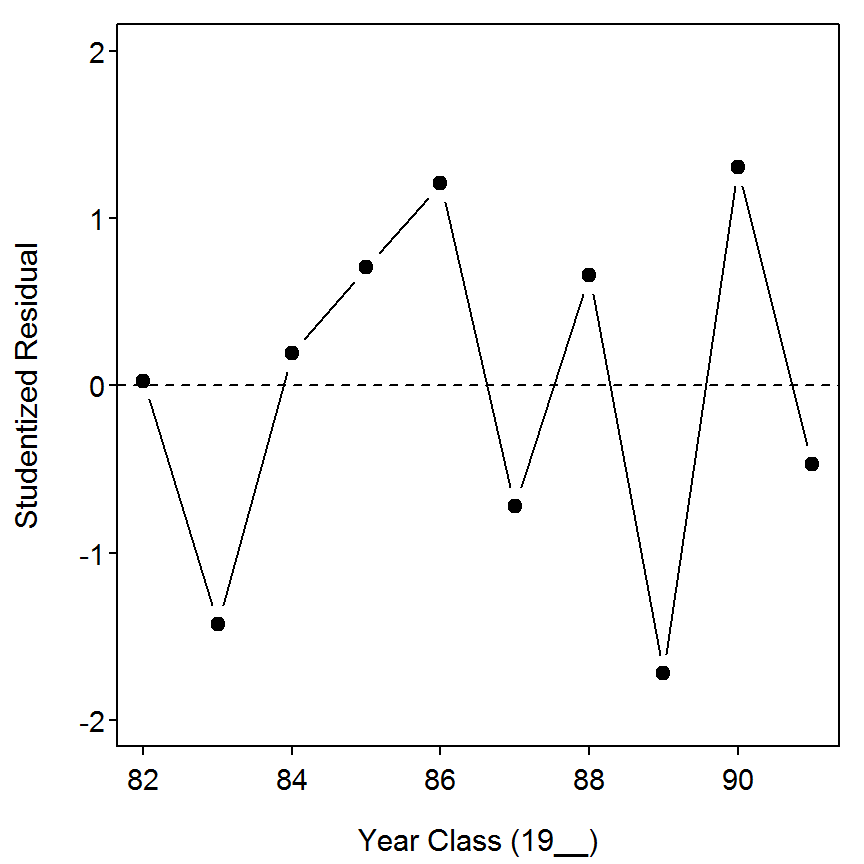> plot(sresid~yearcl,data=ycs,type="h",xlab="Year Class (19__)", ylab="Studentized Residual", pch=19,ylim=c(-2,2),lwd=3) > abline(h=0,lty=2)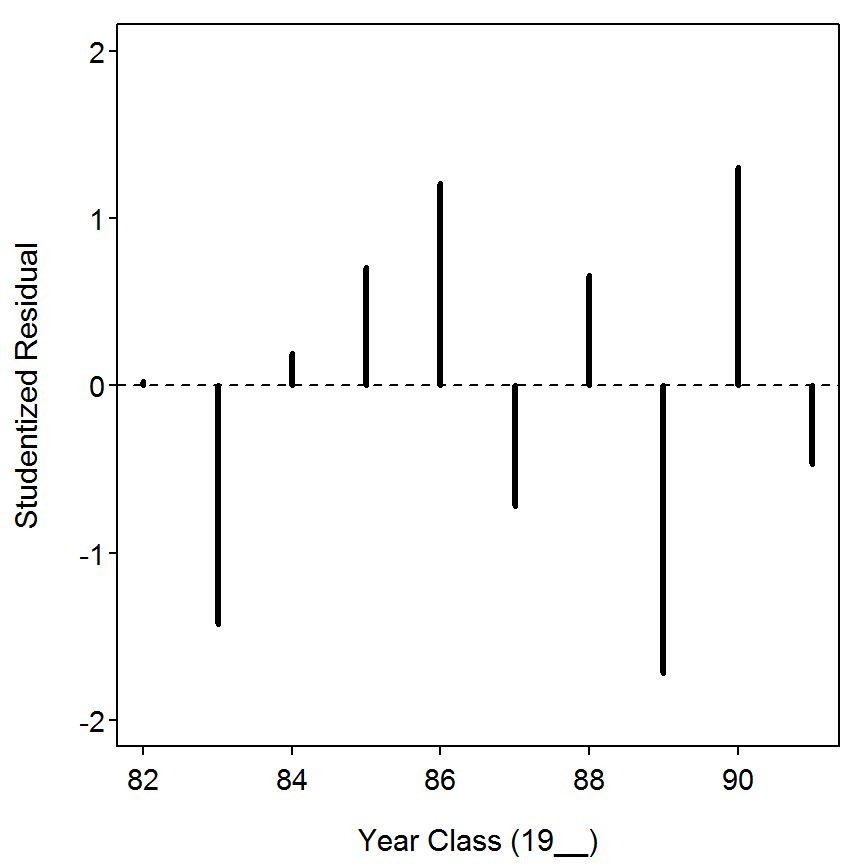## 4.5 Use of Correlation, Simple Regression, and Multiple Regression Analyses to Explain Recruitment Variation From the data presented in Table 4.1 (in text), the relations between C/f age-0 crappies (Pomoxis spp.) (cpe0) and reservoir hydrologic conditions were determined. The respective year-classes (yearcl) were also noted. The following R code plots bivariate relations between C/f of age-0 fish and hydrologic variables, computes the Pearson product moment correlation coefficients among age-0 catch and the reservoir hydrologic terms, and finally computes multiple regressions to describe and predict age-0 catch from these hydrologic variables. ### 4.5.1 Preparing Data The box4_5.txt data file is read and the structure of the data frame and the data frame is observed below. > d5 <- read.table("data/box4_5.txt",header=TRUE) > str(d5) 'data.frame': 11 obs. of 6 variables:$ yearcl  : int  1989 1990 1991 1992 1993 1994 1995 1996 1997 1998 ...
$cpe0 : num NA 8.03 0.47 0.61 1.38 2.73 1.66 9.89 1.86 3.72 ...$ cpe1    : num  3.12 5.32 0.39 0.97 3.59 2.61 0.57 8.63 0.93 1.17 ...
$winstage: num 171 172 171 171 171 ...$ winret  : num  7.2 4.2 7.4 6.6 6.2 5.9 6.8 5.5 6.1 5.6 ...
$sprstage: num 172 172 172 172 172 ... > d5  yearcl cpe0 cpe1 winstage winret sprstage 1 1989 NA 3.12 170.85 7.2 171.79 2 1990 8.03 5.32 171.76 4.2 171.75 3 1991 0.47 0.39 170.73 7.4 171.75 4 1992 0.61 0.97 170.67 6.6 171.82 5 1993 1.38 3.59 170.99 6.2 171.77 6 1994 2.73 2.61 170.93 5.9 171.87 7 1995 1.66 0.57 170.88 6.8 171.80 8 1996 9.89 8.63 171.39 5.5 171.85 9 1997 1.86 0.93 170.83 6.1 171.90 10 1998 3.72 1.17 171.04 5.6 171.83 11 1999 2.18 NA 170.77 9.7 171.92 ### 4.5.2 Summary Statistics and Plots The summary statistics table is computed with Summarize(). One can use lapply() as shown below to compute the summary statistics for each variable in a data frame (NOTE: I excluded the yearcl and cpe1 variables from the data frame for these summaries). > lapply(as.list(d5[,-c(1,3)]),Summarize,digits=4) $cpe0
n   nvalid     mean       sd      min       Q1   median       Q3
11.0000  10.0000   3.2530   3.1838   0.4700   1.4500   2.0200   3.4720
max percZero
9.8900   0.0000

$winstage n nvalid mean sd min Q1 median Q3 11.0000 11.0000 170.9855 0.3216 170.7000 170.8000 170.9000 171.0000 max percZero 171.8000 0.0000$winret
n   nvalid     mean       sd      min       Q1   median       Q3
11.0000  11.0000   6.4727   1.3907   4.2000   5.7500   6.2000   7.0000
max percZero
9.7000   0.0000

$sprstage n nvalid mean sd min Q1 median Q3 11.0000 11.0000 171.8227 0.0578 171.8000 171.8000 171.8000 171.9000 max percZero 171.9000 0.0000  The most efficient way to construct the plots described in the box is with pairs(). The pairs() function requires a formula as its first argument which must start with a tilde followed by the apparent summation of all numeric variables. The data frame in which the variables are found is included in the data= argument. > pairs(~cpe0+winstage+winret+sprstage,data=d5,pch=19)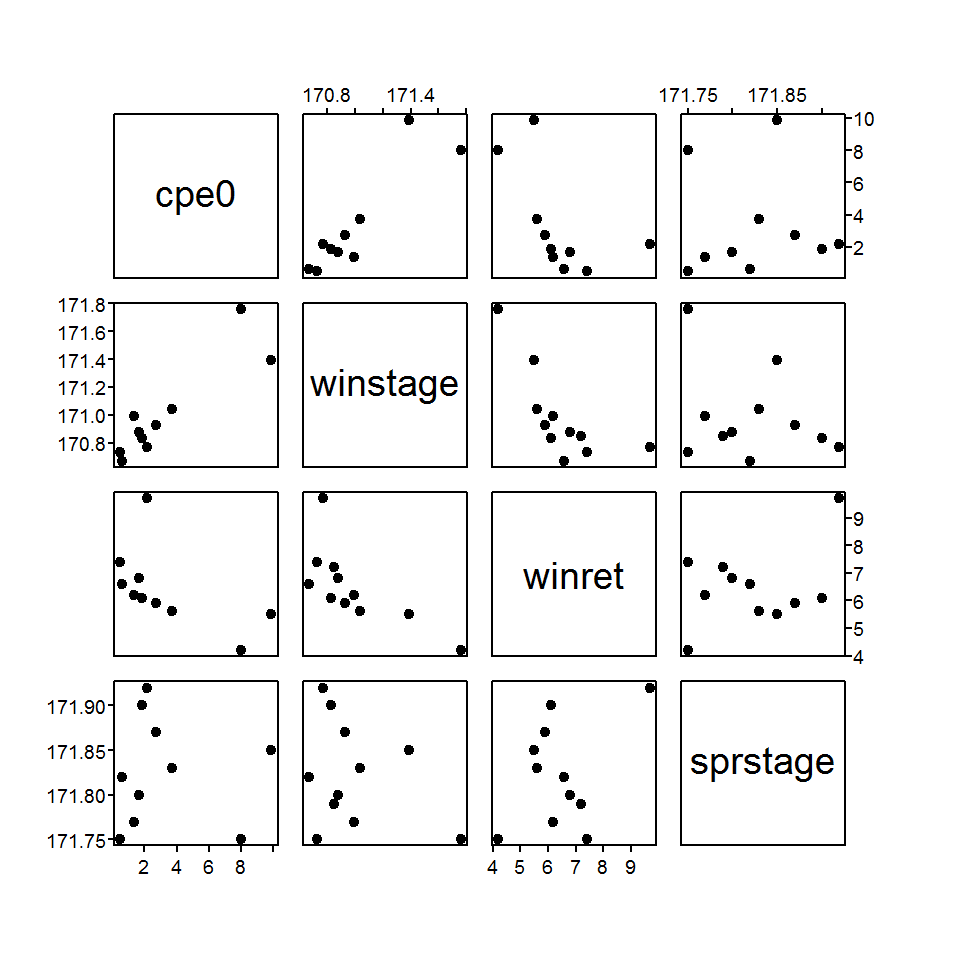From this analysis it appears that the CPE of age-0 fish is highly linearly related to mean winter stage level and mean winter retention (though, this may be curvilinear) and rather weakly related to mean spring stage (though, two potential outliers are evident). In addition, mean winter stage level and mean winter retention appear to be highly negatively correlated (though, potentially curvilinear). ### 4.5.3 Correlation Analysis The authors examined the correlations, with p-values corresponding to a test of whether the correlation is equal to zero, between all variables. This computation is accomplished with rcorr() as described in Box 4.2. > rcorr(as.matrix(d5[,c("cpe0","winstage","winret","sprstage")]))  cpe0 winstage winret sprstage cpe0 1.00 0.89 -0.56 -0.03 winstage 0.89 1.00 -0.72 -0.29 winret -0.56 -0.72 1.00 0.38 sprstage -0.03 -0.29 0.38 1.00 n cpe0 winstage winret sprstage cpe0 10 10 10 10 winstage 10 11 11 11 winret 10 11 11 11 sprstage 10 11 11 11 P cpe0 winstage winret sprstage cpe0 0.0005 0.0902 0.9297 winstage 0.0005 0.0126 0.3820 winret 0.0902 0.0126 0.2485 sprstage 0.9297 0.3820 0.2485  ### 4.5.4 Multiple Linear Regressions The multiple linear regression with three explanatory variables is fit with lm() and saved to an object. The parameter estimates and overall F test statistic are extracted from the saved linear model with summary(). The variance-inflation-factors (VIFs) are extracted with vif() from the car package. The large VIFs are not surprising given the high correlation observed between winstage and winret. > lm1 <- lm(cpe0~winstage+winret+sprstage,data=d5) > summary(lm1) Coefficients: Estimate Std. Error t value Pr(>|t|) (Intercept) -4.238e+03 1.512e+03 -2.803 0.03103 winstage 9.615e+00 1.956e+00 4.914 0.00267 winret 8.491e-02 4.752e-01 0.179 0.86407 sprstage 1.511e+01 8.509e+00 1.776 0.12611 Residual standard error: 1.381 on 6 degrees of freedom (1 observation deleted due to missingness) Multiple R-squared: 0.8746, Adjusted R-squared: 0.8119 F-statistic: 13.95 on 3 and 6 DF, p-value: 0.004103  > vif(lm1) winstage winret sprstage 2.036654 2.222351 1.224579  As the authors of the box suggest, winret is excluded from the analysis as it was highly correlated with winstage but winstage was more highly correlated with cpe0. > lm2 <- lm(cpe0~winstage+sprstage,data=d5) > summary(lm2) Coefficients: Estimate Std. Error t value Pr(>|t|) (Intercept) -4273.241 1391.124 -3.072 0.018022 winstage 9.380 1.347 6.963 0.000219 sprstage 15.553 7.556 2.058 0.078563 Residual standard error: 1.282 on 7 degrees of freedom (1 observation deleted due to missingness) Multiple R-squared: 0.874, Adjusted R-squared: 0.8379 F-statistic: 24.27 on 2 and 7 DF, p-value: 0.0007109  Finally, the simple linear regression with just winstage is fit. > lm3 <- lm(cpe0~winstage,data=d5) > summary(lm3) Coefficients: Estimate Std. Error t value Pr(>|t|) (Intercept) -1445.158 257.907 -5.603 0.000508 winstage 8.470 1.508 5.616 0.000501 Residual standard error: 1.519 on 8 degrees of freedom (1 observation deleted due to missingness) Multiple R-squared: 0.7977, Adjusted R-squared: 0.7724 F-statistic: 31.54 on 1 and 8 DF, p-value: 0.0005008  ### 4.5.5 Final Plot The plot at the end of the box can be constructed in parts. The initial plot is constructed with plot() as usual. Year-class labels for each point are added with thigmophobe.labels() from the plotrix package which takes the x- and y-coordinates as the first two arguments and the labels as the third argument. I subtracted 1900 from each value in the yearcl variable so that only the two-digit year would be printed. The thigmophobe.labels() function positions the labels in a manner that reduces the chances of label overlap. The two uses of abline() are used to add a vertical line at the “Full Summer Pool” value and the best-fit line from the regression of age-0 CPE on mean winter stage. > plot(cpe0~winstage,data=d5,pch=19,xlim=c(170.5,172),ylim=c(0,12), xlab="Mean Winter (Jan-Mar) Stage",ylab="Age-0 CPE") > thigmophobe.labels(d5$winstage,d5$cpe0,d5$yearcl-1900,cex=0.8)
> abline(v=171.95,lty=3)
> abline(lm3,lty=2)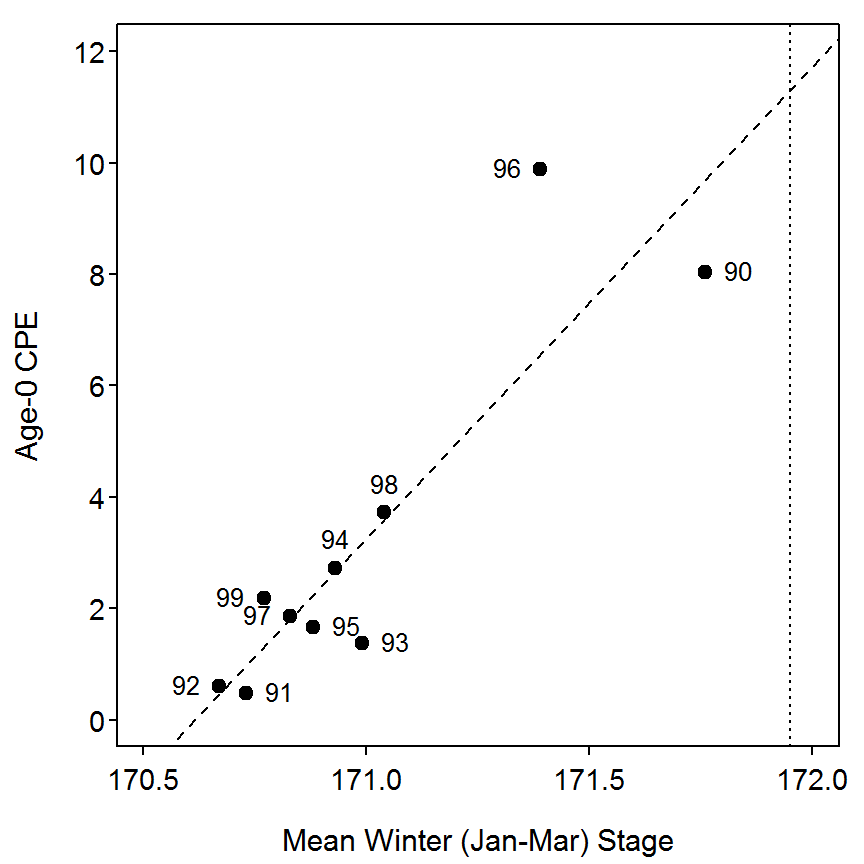## 4.6 Incorporation of an Environmental Term into a Catch-Curve Regression to Explain Fluctuations in Recruitment

From the data presented in the R code in Box 4.4 and the code below, April-July retention will first be plotted against the residuals from the weighted catch-curve regression for largemouth bass. Then, this term will be added to the simple linear catch-curve regression to compute a multiple regression. The mean retention (meanret) between April-July corresponds to the hatching and post-hatching time period for each year-class when fish were age-0 (Maceina et al. 1995).

The same data and ycs data frame from Box 4.4 are used here.

### 4.6.1 Relating Year-Class Strength to Mean Retention Time

The plot of the residuals versus mean retention time (left) and log mean retention time (right) is constructed below. There is a clear curvilinear pattern evident in the raw residuals plot but not in the transformed plot (though a heteroscedasticity is evident). The relatively strong relationship between the residuals from the catch curve model and the log mean retention time suggests that including log mean retention time in the catch curve model may explain a significant portion of the remaining unexplained variability. This term is included in the analysis further below.

> plot(resid~meanret,data=ycs,pch=19,xlab="Mean Retention Time", ylab="Residual")
> abline(h=0,lty=2)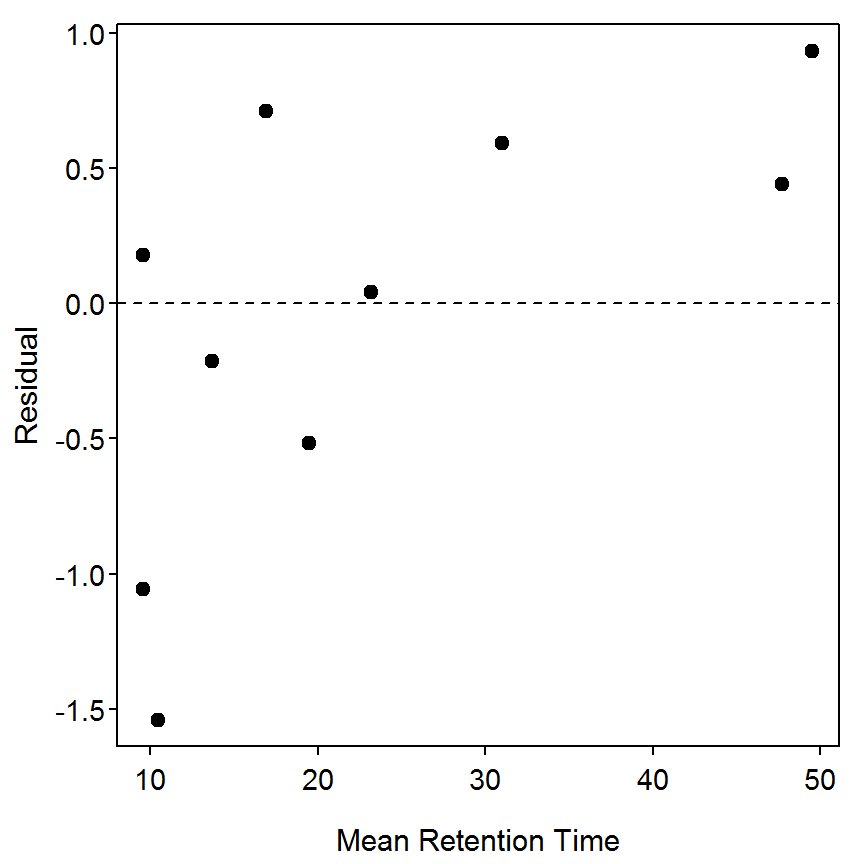> plot(resid~lmeanret,data=ycs,pch=19,xlab="log10 Mean Retention Time", ylab="Residual")
> abline(h=0,lty=2)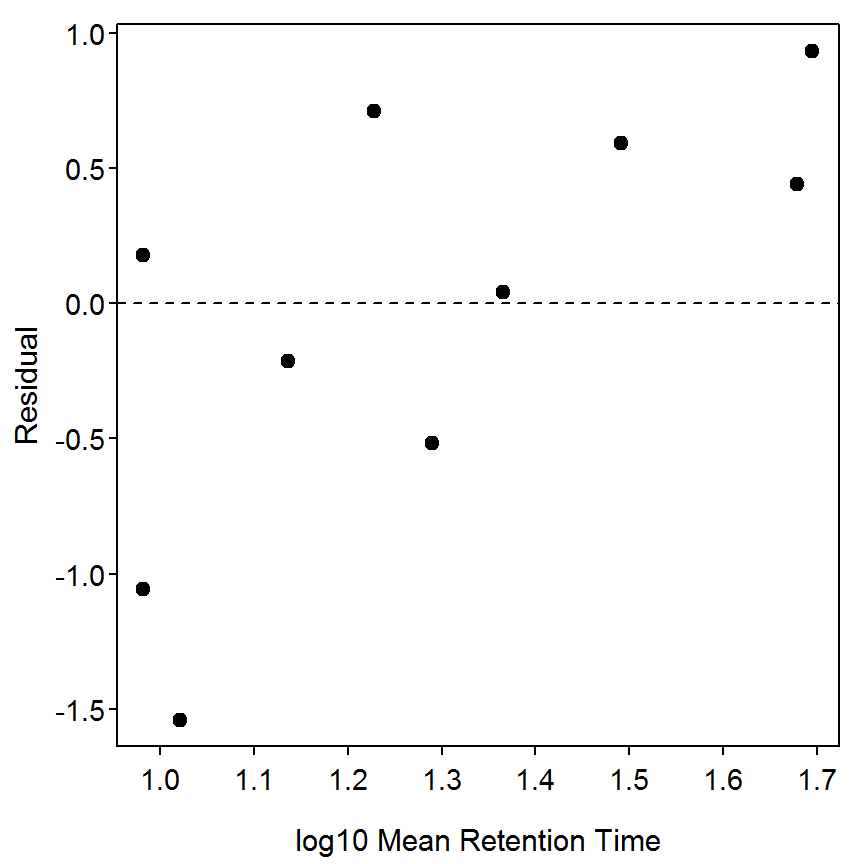The multiple linear regression with the lmeanret variable is computed with lm() by “adding” the lmeanret to the original weighted catch curve model.

> lm3 <- lm(lnum~age+lmeanret,weights=plnum1,data=d4)
> summary(lm3)
Coefficients:
Estimate Std. Error t value Pr(>|t|)
(Intercept)  3.95057    1.00022   3.950 0.005534
age         -0.52606    0.07666  -6.862 0.000239
lmeanret     2.04888    0.77271   2.652 0.032867

Residual standard error: 1.072 on 7 degrees of freedom
Multiple R-squared: 0.8736, Adjusted R-squared: 0.8375
F-statistic:  24.2 on 2 and 7 DF,  p-value: 0.0007173 

The summary statistics and correlation analyses shown in the box are constructed with

> Summarize(ycs$resid,digits=4)  n nvalid mean sd min Q1 median Q3 10.0000 10.0000 -0.0437 0.7975 -1.5390 -0.4411 0.1084 0.5529 max percZero 0.9310 0.0000  > Summarize(ycs$meanret,digits=4)
       n   nvalid     mean       sd      min       Q1   median       Q3
10.0000  10.0000  23.1200  15.0095   9.6000  11.3000  18.2000  29.0500
max percZero
49.5000   0.0000 
> rcorr(cbind(ycs$resid,d4$meanret))
     [,1] [,2]
[1,] 1.00 0.66
[2,] 0.66 1.00

n= 10

P
[,1]  [,2]
[1,]       0.039
[2,] 0.039      

## 4.7 Computation of the Beverton-Holt Recruit-Spawning Curve

From 1991 to 1996, crappies (Pomoxis spp.) were collected from three Alabama reservoirs (lake) that displayed similar hydrologic conditions (data from Ozen (1997)); 16 to 20 trap nets were used as described in Box 4.1. Fish were collected in the fall of each year, aged, and weighed (within 1 g). The variable spawner was determined by dividing total weight of all age-2 and older crappies (assumed to be adults) by the number of net-nights of effort and recruit was determined by dividing the total number of age-0 crappies by the number of net-nights of effort. The code below plots the relation between recruits and spawners, then describes the relations between recruits and spawners using nonlinear regression for untransformed and natural log transformed data (equations [4.3] and [4.5] in the text, respectively). From the last nonlinear regression, predicted recruits (pred.lrec) was regressed against observed recruits to provide additional statistical inference. The predicted number of recruits and associated residuals from the last nonlinear regression were derived and printed. In the nonlinear procedure in R, the parameters statement refers to approximate coefficients for $$\alpha$$ (a in R) and $$\beta$$ (b in R) in the nonlinear regression that are provided by the fisheries scientist to initiate the analysis. Hougaard’s skewness values for $$\alpha$$ and $$\beta$$ were computed for each nonlinear regression. Finally, residual values from the last nonlinear regression were summed.

### 4.7.1 Preparing Data

The box4_7.txt data file is read and the structure of the data frame is observed below. In addition, the authors of the box created a new variable that contains the natural log of the number of recruits.

> d7 <- read.table("data/box4_7.txt",header=TRUE)
> str(d7)
'data.frame':   18 obs. of  4 variables:
$lake : Factor w/ 3 levels "AL","DE","JB": 1 1 1 1 1 1 2 2 2 2 ...$ year   : int  91 92 93 94 95 96 92 93 94 95 ...
$spawner: int 340 907 171 1040 55 524 213 1034 457 200 ...$ recruit: num  5.41 3 2.41 2.25 0.41 8.71 1.13 4.66 1.94 7.28 ...
> d7$lrecruit <- log(d7$recruit)

### 4.7.2 Plot of Stock-Recruit Data

The plot, mentioned but not shown in the box, is constructed as shown below.

> plot(recruit~spawner,data=d7,ylab="Catch Rate of Age-0 Crappie",
xlab="Biomass of Age-2+ Crappie",pch=19)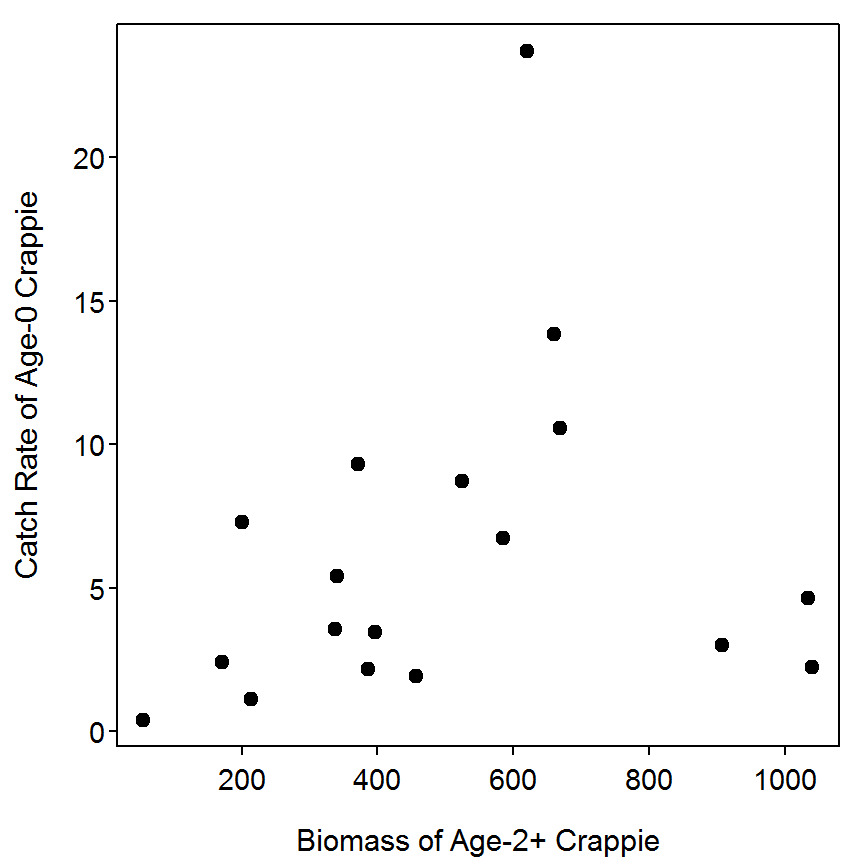### 4.7.3 Non-Linear Model Fit with Additive Errors (i.e., Raw Data)

The non-linear model fitting procedure in R is implemented with nls(), which requires the model formula, the list of starting values, and the data frame containing the variables as arguments. In addition, trace=TRUE can be included in nls() to see the residual sum-of-squares and current values of the parameters for each iteration of the fitting process. For simplicity and clarity, the starting values can be entered into a list and the formula corresponding to the Beverton-Holt stock-recruit model are created prior to calling nls(). The parameter estimates and correlation among parameters are extracted from the saved nls() object with overview() from the nlstools package.

> bhst <- list(a=0.03,b=0.002)                     # list of starting values
> bhsr <- recruit~(a*spawner)/(1+b*spawner)        # B-H model as an R formula
> nls1 <- nls(bhsr,data=d7,start=bhst,trace=TRUE)
502.8573 :  0.030 0.002
486.2032 :  0.041785368 0.003879117
484.4992 :  0.037706303 0.003577408
484.485 :  0.039117234 0.003767486
484.4819 :  0.038257852 0.003643063
484.4802 :  0.038831928 0.003725968
484.4796 :  0.038454230 0.003671472
484.4793 :  0.038704677 0.003707628
484.4792 :  0.038539434 0.003683781
484.4791 :  0.038648837 0.003699574
484.4791 :  0.038576567 0.003689143
484.4791 :  0.038624375 0.003696044
484.4791 :  0.038592774 0.003691483
484.4791 :  0.03861368 0.00369450
484.4791 :  0.038599860 0.003692506
484.4791 :  0.038608998 0.003693825
484.4791 :  0.038602954 0.003692952
484.4791 :  0.038606952 0.003693529
484.4791 :  0.038604309 0.003693148
> overview(nls1)

------
Formula: recruit ~ (a * spawner)/(1 + b * spawner)

Parameters:
Estimate Std. Error t value Pr(>|t|)
a 0.038604   0.047423   0.814    0.428
b 0.003693   0.006752   0.547    0.592

Residual standard error: 5.503 on 16 degrees of freedom

Number of iterations to convergence: 18
Achieved convergence tolerance: 9.341e-06

------
Residual sum of squares: 484

------
t-based confidence interval:
2.5%      97.5%
a -0.06192736 0.13913598
b -0.01062148 0.01800778

------
Correlation matrix:
a         b
a 1.0000000 0.9864924
b 0.9864924 1.0000000

### 4.7.4 Non-Linear Model Fit with Multiplicative Errors (i.e., Log Transformed Data)

The Beverton-Holt model with multiplicative errors is fit similarly with the only major adjustment being that the both sides of the model are log-transformed.

> bhst2 <- list(a=0.01,b=0.002)
> bhsr2 <- lrecruit~log((a*spawner)/(1+b*spawner))
> nls2 <- nls(bhsr2,data=d7,start=bhst2)
> overview(nls2)

------
Formula: lrecruit ~ log((a * spawner)/(1 + b * spawner))

Parameters:
Estimate Std. Error t value Pr(>|t|)
a 0.016980   0.009339   1.818   0.0878
b 0.001423   0.001918   0.742   0.4688

Residual standard error: 0.7957 on 16 degrees of freedom

Number of iterations to convergence: 10
Achieved convergence tolerance: 7.649e-06

------
Residual sum of squares: 10.1

------
t-based confidence interval:
2.5%       97.5%
a -0.002818814 0.036778136
b -0.002642872 0.005489538

------
Correlation matrix:
a         b
a 1.0000000 0.9400708
b 0.9400708 1.0000000

### 4.7.5 Bootstrapping Confidence Intervals

The nlsBoot() from the nlstools package is used to bootstrap the residuals from a non-linear model fit. This function only requires the model as an argument, but the number of bootstrap samples can be controlled with the niter= argument. The mean parameter values and confidence intervals are constructed from the saved nlsBoot object using summary().

> bhbc <- nlsBoot(nls2,niter=2000)
> summary(bhbc)

------
Bootstrap statistics
Estimate Std. error
a 0.027006261 0.04774726
b 0.003720517 0.01088564

------
Median of bootstrap estimates and percentile confidence intervals
Median         2.5%      97.5%
a 0.018311724 8.933571e-03 0.08917533
b 0.001691925 4.157789e-05 0.01744845

### 4.7.6 Diagnostics

The predicted number of log recruits from the multiplicative errors model is computed with fitted() and the residuals are computed with residuals(). These two items are appended to the data frame and the residuals are summed below.

> d7$pred.lrec <- fitted(nls2) > d7$res.lrec <- residuals(nls2)
> d7
   lake year spawner recruit   lrecruit  pred.lrec    res.lrec
1    AL   91     340    5.41  1.6882491  1.3585103  0.32973876
2    AL   92     907    3.00  1.0986123  1.9054310 -0.80681870
3    AL   93     171    2.41  0.8796267  0.8480830  0.03154377
4    AL   94    1040    2.25  0.8109302  1.9628708 -1.15194063
5    AL   95      55    0.41 -0.8915981 -0.1437761 -0.74782197
6    AL   96     524    8.71  2.1644718  1.6285244  0.53594735
7    DE   92     213    1.13  0.1222176  1.0207533 -0.89853571
8    DE   93    1034    4.66  1.5390154  1.9605340 -0.42151858
9    DE   94     457    1.94  0.6626880  1.5478883 -0.88520030
10   DE   95     200    7.28  1.9851309  0.9720790  1.01305184
11   DE   96     669   10.56  2.3570733  1.7610829  0.59599034
12   JB   90     372    9.33  2.2332350  1.4182270  0.81500803
13   JB   91     386    2.19  0.7839015  1.4422262 -0.65832470
14   JB   92     585    6.75  1.9095425  1.6901098  0.21943273
15   JB   93     660   13.85  2.6282852  1.7541221  0.87416311
16   JB   94     337    3.58  1.2753628  1.3525293 -0.07716648
17   JB   95     396    3.48  1.2470323  1.4586587 -0.21162642
18   JB   96     620   23.70  3.1654750  1.7213974  1.44407761
> sum(d7$res.lrec)  2.254291e-08 The simple linear regression of the predicted number of log recruits on the observed number of log recruits is fit and the anova table and coefficients are extracted below. > lm1 <- lm(pred.lrec~lrecruit,data=d7) > anova(lm1)  Df Sum Sq Mean Sq F value Pr(>F) lrecruit 1 1.6680 1.66803 9.7105 0.006651 Residuals 16 2.7484 0.17178 Total 17 4.4164  > summary(lm1) Coefficients: Estimate Std. Error t value Pr(>|t|) (Intercept) 0.9662 0.1768 5.464 5.2e-05 lrecruit 0.3222 0.1034 3.116 0.00665 Residual standard error: 0.4145 on 16 degrees of freedom Multiple R-squared: 0.3777, Adjusted R-squared: 0.3388 F-statistic: 9.71 on 1 and 16 DF, p-value: 0.006651  ### 4.7.7 Final Fitted Plot Finally, a plot that shows the raw data with fitted lines from the two non-linear fits can be constructed as shown below. In this code, a plot of the data is constructed first, the coefficients of the first model are extracted with coef() and saved, and those coefficients are used in curve() to add the model curve on to the plot. This is repeated for the second model and a legend is added in the top-left corner of the plot. > plot(recruit~spawner,data=d7,pch=19) > cnls1 <- coef(nls1) > curve((cnls1*x)/(1+cnls1*x),from=min(d7$spawner),to=max(d7$spawner),col="red", lwd=2,lty=2,add=TRUE) > cnls2 <- coef(nls2) > curve((cnls2*x)/(1+cnls2*x),from=min(d7$spawner),to=max(d7$spawner),col="blue", lwd=2,lty=2,add=TRUE) > legend("topleft",legend=c("Additive Error","Multiplicative Error"),col=c("red","blue"), lwd=2,lty=2,cex=0.5)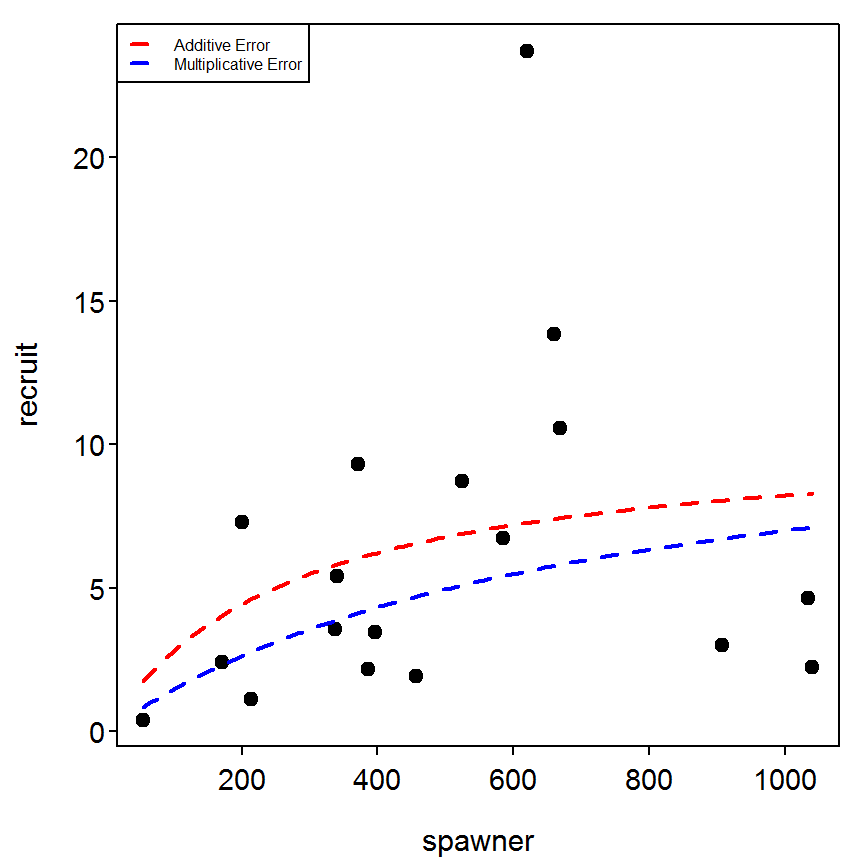## 4.8 Computation of Ricker Recruit-Spawner Curves with the Inclusion of an Environmental Term to Explain Recruit Variation Population estimates for age-5 and older adult walleye (Sander vitreus) (spawner) and age-0 walleye (recruit) were made in Escanaba Lake, Wisconsin, from 1958 to 1991 (data from Hansen et al. (1998); see Table 4.3 in text). The following R code computes a nonlinear regression to describe the relation between recruits and spawners assuming lognormal error structure (equation [4.6] in text). From this regression, predicted recruits are regressed against observed recruits to provide additional statistical inference. Next the program computes the Ricker recruit-spawner relation (equation [4.7] in text) using linear regression. The corrected coefficient of determination and associated F-statistic was given by regressing predicted recruits against observed recruits. Finally, the program also computes the nonlinear regression with lognormal error structure in the recruit-spawner relation to include the variation in May air temperature (mtempcv) as an additional regressor of walleye recruits (equation [4.9] in text modified to include lognormal error structure). ### 4.8.1 Preparing Data The box4_8.txt data file is read and the structure of the data frame is observed below. The analyses below require a variable that is the natural log of the number of recruits, natural log of the number of spawners, the ratio of recruits to spawners, and the log of this ratio. These variables are created and added to the data frame. > d8 <- read.table("data/box4_8.txt",header=TRUE) > str(d8) 'data.frame': 34 obs. of 4 variables:$ year   : int  1958 1959 1960 1961 1962 1963 1964 1965 1966 1967 ...
$recruit: int 4532 22996 628 879 14747 13205 31793 10621 22271 8736 ...$ spawner: int  775 2310 2990 1400 1130 790 1195 981 870 1104 ...
$mtempcv: num 0.241 0.163 0.461 0.33 0.226 ... > d8$logR <- log(d8$recruit) > d8$logS <- log(d8$spawner) > d8$ratio <- d8$recruit/d8$spawner
> d8$lratio <- log(d8$ratio)
> view(d8)
   year recruit spawner mtempcv      logR     logS     ratio    lratio
7  1964   31793    1195 0.19229 10.367001 7.085901 26.605021 3.2811000
12 1969   18885    1421 0.17799  9.846123 7.259116 13.289937 2.5870071
15 1972    1697    1354 0.39461  7.436617 7.210818  1.253323 0.2257988
18 1975    1932     962 0.33459  7.566311 6.869014  2.008316 0.6972966
21 1978    5334    1945 0.32837  8.581857 7.573017  2.742416 1.0088394
28 1985   14599     394 0.12269  9.588708 5.976351 37.053299 3.6123574

### 4.8.2 Plot of Stock-Recruit Data

I wanted to visualize the stock-recruit relationship before fitting any models in two different ways. I first plotted the stock-recruit plot with year labels next to each point. The year labels for each point are added with thigmophobe.labels() as described in Box 4.5. I then plotted the stock-recruit plot but with the size of each plotted point relative to the value of the mtempcv variable. This plotting requires use of the “character expansion” (i.e., cex=) argument in plot(). The default character size is 1 such that, for example, a cex=2 value would produce a point twice as big as typical. I rescaled (with rescale() from the plotrix package) the mtempcv variable to take values between 0.5 and 2 so that relatively small values of mtempcv would produce smaller points and relatively larger values of mtempcv would produce larger points. It is apparent from these plots that the stock-recruit relationship is weak and that there may be a very weak relationship with the variation in May temperatures (it seems that lower recruitment may correspond to higher temperature variability).

> plot(recruit~spawner,data=d8,ylab="Number of Age-0 Walleye",
xlab="Number of Age-5 Walleye",pch=19)
> with(d8,thigmophobe.labels(spawner,recruit,labels=year-1900,cex=0.8))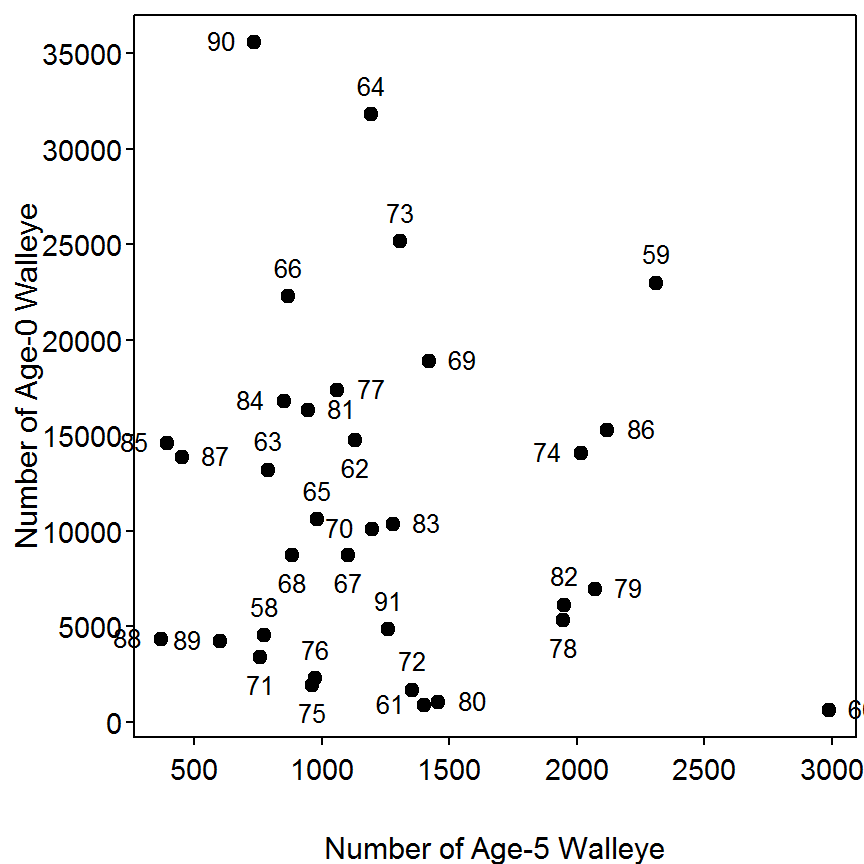> plot(recruit~spawner,data=d8,ylab="Number of Age-0 Walleye",
xlab="Number of Age-5 Walleye",pch=19,cex=rescale(mtempcv,c(0.5,2)))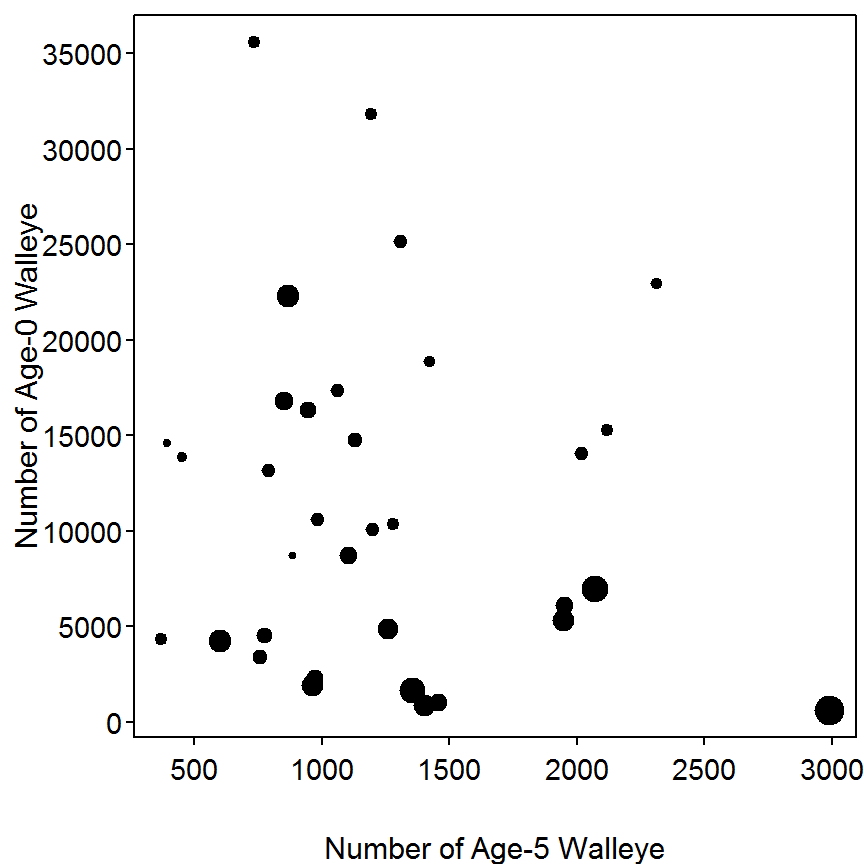### 4.8.3 Ricker Model - Nonlinear Regression with Multiplicative Errors

The non-linear model fitting procedure in R is implemented with nls() as described in Box 4.7. The model is fit with multiplicative errors if both the sides of the formula are log-transformed.

> rst <- list(a=4,b=0)                           # Starting values
> rsr <- logR~log(spawner*exp(a+b*spawner))      # Ricker model as an R formula
> nls1 <- nls(rsr,data=d8,start=rst,trace=TRUE)
193.9594 :  4 0
33.79315 :   3.391569209 -0.001176262
> overview(nls1)

------
Formula: logR ~ log(spawner * exp(a + b * spawner))

Parameters:
Estimate Std. Error t value Pr(>|t|)
a  3.3915692  0.4117562   8.237 2.08e-09
b -0.0011763  0.0003018  -3.898 0.000467

Residual standard error: 1.028 on 32 degrees of freedom

Number of iterations to convergence: 1
Achieved convergence tolerance: 5.208e-10

------
Residual sum of squares: 33.8

------
t-based confidence interval:
2.5%         97.5%
a  2.552849366  4.2302890519
b -0.001790988 -0.0005615366

------
Correlation matrix:
a          b
a  1.0000000 -0.9037713
b -0.9037713  1.0000000

The predicted number of log recruits and the residuals from the multiplicative errors model are appended to the original data frame and the sum of the residuals is shown to be equal to zero.

> d8$pred1.lrec <- fitted(nls1) > d8$res1.lrec <- residuals(nls1)
> sum(d8$res1.lrec)  -4.438914e-08 The simple linear regression of the predicted number of log recruits on the observed number of log recruits is fit with lm() and summary() is used to extract the model coefficients and, as illustrated in the box, the $$R^{2}$$ value for the non-linear model fit. > lm1 <- lm(pred1.lrec~logR,data=d8) > summary(lm1) Coefficients: Estimate Std. Error t value Pr(>|t|) (Intercept) 8.35871 0.40507 20.635 <2e-16 logR 0.06577 0.04498 1.462 0.153 Residual standard error: 0.27 on 32 degrees of freedom Multiple R-squared: 0.06263, Adjusted R-squared: 0.03334 F-statistic: 2.138 on 1 and 32 DF, p-value: 0.1534  ### 4.8.4 Ricker Model – Linear Regression The Ricker model can be linearized via transformation as described in the AIFFD book. This linearized version of the model is fit and summarized below. The predicted number of log recruits from the linear model is a bit more difficult to obtain because this model predicts the log ratio of recruits to spawners. Thus, these predictions should be back-transformed to the original scale (i.e., the ratio), multiplied by the number of spawners to get the predicted number of recruits, and then logged to get the predicted log number of recruits. This process of computing predicted log number of recruits is shown below followed by the regression of of these predictions on the observed log recruits and the summary of that model fit. > lm2 <- lm(lratio~spawner,data=d8) > summary(lm2) Coefficients: Estimate Std. Error t value Pr(>|t|) (Intercept) 3.3915692 0.4117562 8.237 2.08e-09 spawner -0.0011763 0.0003018 -3.898 0.000467 Residual standard error: 1.028 on 32 degrees of freedom Multiple R-squared: 0.3219, Adjusted R-squared: 0.3007 F-statistic: 15.19 on 1 and 32 DF, p-value: 0.0004665  > d8$pred2.lrec <- log(exp(fitted(lm2))*d8$spawner) > lm2a <- lm(pred2.lrec~logR,data=d8) > summary(lm2a) Coefficients: Estimate Std. Error t value Pr(>|t|) (Intercept) 8.35871 0.40507 20.635 <2e-16 logR 0.06577 0.04498 1.462 0.153 Residual standard error: 0.27 on 32 degrees of freedom Multiple R-squared: 0.06263, Adjusted R-squared: 0.03334 F-statistic: 2.138 on 1 and 32 DF, p-value: 0.1534  ### 4.8.5 Ricker Model with Environmental Component - Nonlinear Regression with Lognormal Errors In this analysis the authors return to the original nonlinear model but include the variation in May temperatures variable (i.e., mtempcv) as another potential prediction of the number of recruits. The model fit and summary extraction is essential as before with the exception that there is an additional variable and parameter in the model. > rst2 <- list(a=4,b=0,c=-7) > rsr2 <- logR~log(spawner*exp(a+b*spawner+c*mtempcv)) > nls2 <- nls(rsr2,data=d8,start=rst2,trace=TRUE) 32.5687 : 4 0 -7 21.99897 : 4.7915401817 -0.0007299359 -7.8388472553 > overview(nls2)  ------ Formula: logR ~ log(spawner * exp(a + b * spawner + c * mtempcv)) Parameters: Estimate Std. Error t value Pr(>|t|) a 4.7915402 0.4815157 9.951 3.6e-11 b -0.0007299 0.0002705 -2.698 0.011181 c -7.8388473 1.9228196 -4.077 0.000295 Residual standard error: 0.8424 on 31 degrees of freedom Number of iterations to convergence: 1 Achieved convergence tolerance: 4.898e-09 ------ Residual sum of squares: 22 ------ t-based confidence interval: 2.5% 97.5% a 3.809482450 5.7735979130 b -0.001281694 -0.0001781775 c -11.760463746 -3.9172307647 ------ Correlation matrix: a b c a 1.0000000 -0.2907282 -0.7131731 b -0.2907282 1.0000000 -0.4046843 c -0.7131731 -0.4046843 1.0000000 > d8$pred3.lrec <- fitted(nls2)
> d8$res3.lrec <- residuals(nls2) > sum(d8$res2.lrec)
 0
> lm4 <- lm(pred3.lrec~logR,data=d8)
> summary(lm4)
Coefficients:
Estimate Std. Error t value Pr(>|t|)
(Intercept)  5.46530    0.77538   7.049 5.43e-08
logR         0.38916    0.08609   4.520 7.97e-05

Residual standard error: 0.5169 on 32 degrees of freedom
Multiple R-squared: 0.3897, Adjusted R-squared: 0.3706
F-statistic: 20.43 on 1 and 32 DF,  p-value: 7.968e-05 

The relative significance of the mtempcv variable can be assessed by computing the SS explained by using model nls2 as compared to nls1 (this is the idea suggested by the authors of the box when they mentioned Montgomery and Peck (1982)). This comparison is constructed in R by submitting these two non-lm() objects to anova().

> anova(nls1,nls2)
  Res.Df Res.Sum Sq Df Sum Sq F value   Pr(>F)
1     32     33.793
2     31     21.999  1 11.794   16.62 0.000295

In addition, the model with the lowest AIC value is the “best” model. The AICs for both models are extracted by submitting the two non-linear models to nls().

> AIC(nls1,nls2)
     df       AIC
nls1  3 102.28034
nls2  4  89.68541

### 4.8.6 Final Fitted Plot

Finally, a plot that shows the raw data with fitted lines from the non-linear fits without the environmental variable and assuming multiplicative (i.e., nls1) and additive (computed below) errors is constructed below. This code follows the same concept as that described at the end of Box 4.7.

> rsr3 <- recruit~spawner*exp(a+b*spawner)
> nls3 <- nls(rsr3,data=d8,start=rst)
> plot(recruit~spawner,data=d8,pch=19,xlim=c(0,max(d8$spawner))) > cnls1 <- coef(nls1) > curve(x*exp(cnls1+cnls1*x),from=0,to=max(d8$spawner),col="red",lwd=2,lty=2,add=TRUE)
> cnls3 <- coef(nls3)
> curve(x*exp(cnls3+cnls3*x),from=0,to=max(d8$spawner),col="blue",lwd=2,lty=2,add=TRUE) > legend("topright",legend=c("Multiplicative Error","Additive Error"),col=c("red","blue"), lwd=2,lty=2,cex=0.5)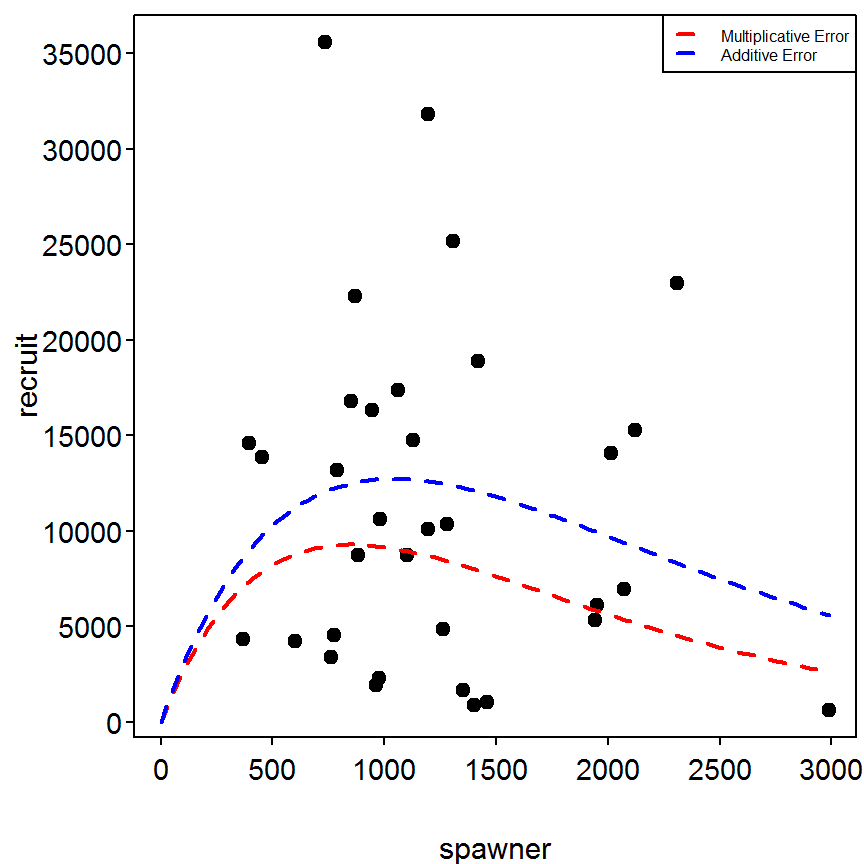## 4.9 Computation of Bootstrapped Parameter Estimates for the Ricker Recruit-Spawner Curve The R code below conducts bootstrapped parameter estimation for walleye (Sander vitreus) recruit-spawner data (recruits given as recruit, spawners given as spawner) listed in Table 4.3 (in text). The code uses the nonlinear form of the Ricker recruit-spawner relation and incorporates lognormal error structure (equation [4.6] in text). In total, 500 estimates were generated. The same data used in Box 4.8 is used in this section. ### 4.9.1 Ricker Model - Nonlinear Regression with Multiplicative Errors The Ricker model with multiplicative errors was fit in Box 4.8. This fitting is repeated below but the specific description of the methodology is not repeated. > d8$logR <- log(d8$recruit) > rst <- list(a=4,b=0) > rsr <- logR~log(spawner*exp(a-b*spawner)) > nls1 <- nls(rsr,data=d8,start=rst) > overview(nls1)  ------ Formula: logR ~ log(spawner * exp(a - b * spawner)) Parameters: Estimate Std. Error t value Pr(>|t|) a 3.3915692 0.4117562 8.237 2.08e-09 b 0.0011763 0.0003018 3.898 0.000467 Residual standard error: 1.028 on 32 degrees of freedom Number of iterations to convergence: 1 Achieved convergence tolerance: 4.887e-09 ------ Residual sum of squares: 33.8 ------ t-based confidence interval: 2.5% 97.5% a 2.5528493665 4.230289052 b 0.0005615366 0.001790988 ------ Correlation matrix: a b a 1.0000000 0.9037713 b 0.9037713 1.0000000 ### 4.9.2 Bootstrapping - Basic Analyses The nlsBoot() function as described in Box 4.7 is used to bootstrap the residuals from a non-linear model fit. The summary() function can be used to extract the median values and 95% confidence intervals for each parameter from the bootstrap samples by submitting just the nlsBoot object. The confint() function extracts just the confidence intervals for each parameter and allows the user to choose a level of confidence with the optional conf.level= argument. > rbc <- nlsBoot(nls1,niter=2000) > summary(rbc)  ------ Bootstrap statistics Estimate Std. error a 3.393918490 0.3937028325 b 0.001175819 0.0002918835 ------ Median of bootstrap estimates and percentile confidence intervals Median 2.5% 97.5% a 3.385565304 2.6296306151 4.163318790 b 0.001167843 0.0006253082 0.001754622 > confint(rbc) # default 95% CI  95% LCI 95% UCI a 2.6296306151 4.163318790 b 0.0006253082 0.001754622 > confint(rbc,conf.level=0.9) # illustrative 90% CI  90% LCI 90% UCI a 2.7458150964 4.036147593 b 0.0007177558 0.001665995 ### 4.9.3 Bootstrapping - Further Analyses The parameter estimates for each bootstrap sample are contained in the coefboot matrix object of the saved nlsBoot() object as illustrated below. > str(rbc) List of 4$ coefboot: num [1:2000, 1:2] 3.39 2.95 4.64 3.86 3.5 ...
..- attr(*, "dimnames")=List of 2
.. ..$: NULL .. ..$ : chr [1:2] "a" "b"
$rse : num [1:2000] 1.029 0.821 0.873 1.064 0.959 ...$ bootCI  : num [1:2, 1:3] 3.385565 0.001168 2.629631 0.000625 4.163319 ...
..- attr(*, "dimnames")=List of 2
.. ..$: chr [1:2] "a" "b" .. ..$ : chr [1:3] "Median" "2.5%" "97.5%"
$estiboot: num [1:2, 1:2] 3.393918 0.001176 0.393703 0.000292 ..- attr(*, "dimnames")=List of 2 .. ..$ : chr [1:2] "a" "b"
.. ..$: chr [1:2] "Estimate" "Std. error" - attr(*, "class")= chr "nlsBoot" > view(rbc$coefboot)
            a           b
[1,] 3.996118 0.001726619
[2,] 3.506436 0.001433546
[3,] 3.315255 0.001219446
[4,] 3.407417 0.001183765
[5,] 2.853613 0.000803138
[6,] 2.464638 0.000492226

Thus, the parameter values from each bootstrap sample can be accessed in order to form a variety of summaries. These values are slightly easier to access if the coefboot object in the nlsboot object is saved as a data frame using as.data.frame(). With this, the summary statistics for a parameter are found with summary() and one-sample t-tests of whether the bootstrapped mean of the parameter equals zero or not is computer with t.test().

> rbc.d <- as.data.frame(rbc$coefboot) > Summarize(rbc.d$a)
           n       nvalid         mean           sd          min           Q1
2000.0000000 2000.0000000    3.3939185    0.3937028    2.1270000    3.1320000
median           Q3          max     percZero
3.3860000    3.6750000    4.6420000    0.0000000 
> Summarize(rbc.d$b)  n nvalid mean sd min Q1 median 2.0000e+03 2.0000e+03 1.1758e-03 2.9190e-04 2.8930e-04 9.6920e-04 1.1680e-03 Q3 max percZero 1.3790e-03 2.1590e-03 0.0000e+00  > t.test(rbc.d$a)
One Sample t-test with rbc.d$a t = 385.5208, df = 1999, p-value < 2.2e-16 alternative hypothesis: true mean is not equal to 0 95 percent confidence interval: 3.376654 3.411183 sample estimates: mean of x 3.393918  > t.test(rbc.d$b)
One Sample t-test with rbc.d$b t = 180.1549, df = 1999, p-value < 2.2e-16 alternative hypothesis: true mean is not equal to 0 95 percent confidence interval: 0.001163019 0.001188619 sample estimates: mean of x 0.001175819  The quantiles presented in the box are constructed with quantile() include the vector of probabilities in the probs= argument. > quantile(rbc.d$a,probs=c(0,1,5,10,25,50,75,90,95,99,100)/100)
      0%       1%       5%      10%      25%      50%      75%      90%
2.127056 2.464483 2.745815 2.887022 3.131924 3.385565 3.674882 3.902363
95%      99%     100%
4.036148 4.328816 4.642484 
> quantile(rbc.d$b,probs=c(0,1,5,10,25,50,75,90,95,99,100)/100)  0% 1% 5% 10% 25% 50% 0.0002893182 0.0005091121 0.0007177558 0.0008084656 0.0009691694 0.0011678427 75% 90% 95% 99% 100% 0.0013792831 0.0015567731 0.0016659945 0.0018392287 0.0021594678  Histograms, with some modifications, of a set of parameter estimates is created with hist(). > hist(rbc.d$a,xlab="Bootstrap Estimates of a",main="")
> abline(v=coef(nls1)["a"],lty=2,lwd=2,col="red")       # put nls estimate on hist
> abline(